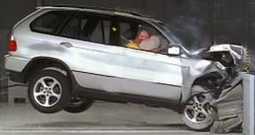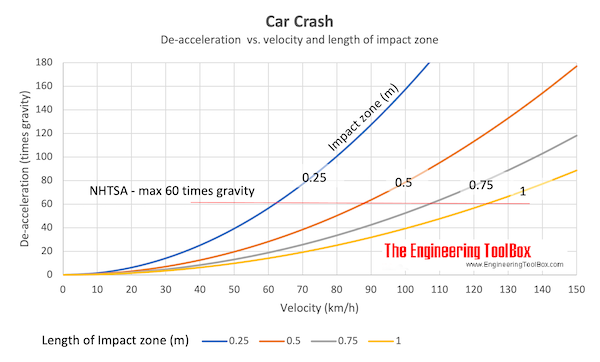Engineering ToolBox - Resources, Tools and Basic Information for Engineering and Design of Technical Applications!

# Impact Force

## Impact forces acting on falling objects hitting the ground, cars crashing and similar cases.The dynamic kinetic energy of a moving object, like a falling ball or a driving car, can be expressed as

E = 1/2 m v2               (1)

where

E = kinetic (dynamic) energy (J, ft lb)

m = mass of the object (kg, slugs)

v = velocity of the object (m/s, ft/s)

In an impact - like a car crash - the work made by the impact force slowing down an moving object over a distance by deforming the crumple zone can be expressed as

W = Favg s                     (2)

where

W = work done (J, ft lb)

Favg = average impact force during deformation (N, lbf)

s = deformation distance, crumple zone (m, ft)

When a crumple zone deforms in a car crash the average impact force is designed to be as constant as possible.

In an impact where the object is not deformed -  the work made by the impact force slowing down the moving object equals to the work done by a spring force - and can be expressed as

W = 1/2 Fmax s

= 1/2 k s2               (2b)

where

W = work done (J, ft lb)

Fmax = maximum force at the end of the deformation (N, lbf)

k = spring constant

s = deformation distance (m, ft)

In a car crash the dynamic energy is converted to work and equation 1 and 2  can be combined to

Favg s = 1/2 m v2                (3)

The average impact force can be calculated as

Favg = 1/2 m v2 / s                  (3b)

The deformation slow-down distance can be calculated as

s = 1/2 m v2 Favg                (3c)

Note! - The deformation slow-down distance is very important and the key to limit the forces acting on passengers in a car crash.

### Example - Car Crash

A car with a mass of 2000 kg drives with speed 60 km/h (16.7 m/s) before it crashes into a massive concrete wall. The front of the car impacts 0.5 m (the deformation distance).

The impact force can be calculated as

Fmax = 1/2 (2000 kg) (16.7 m/s)2 / (0.5 m)

= 558 kN

Note that the gravitation force (weight) acting on the car is only

Fw = m g

= (2000 kg) (9.81 m/s2)

= 19.6 kN

The impact creates a force 28 times gravity!!

A person sitting inside the car with seat belts on will de-accelerate with a force 28 times gravity. Note that the National Highway Traffic Safety Administration (NHTSA) states that "the maximum chest acceleration shall not exceed 60 times gravity for time periods longer than 3 milliseconds". For a car crash with 90 km/h (25 m/s) the de-acceleration will be 64 times gravity (same parameters as above).• 60 mph = 96.6 km/h

### Impact Force from a Falling Object

The dynamic energy in a falling object at the impact moment when it hits the ground can be calculated as

E = Fweight h

= m ag h              (4)

where

Fweight = force due to gravity - or weight (N, lbf)

ag = acceleration of gravity (9.81 m/s2, 32.17405 ft/s2)

h = falling height (m)

If the dynamic energy from the fall is converted to impact work - equation 2 and 4 can be combined to

Favg s = m ag h                      (5)

The impact force can be expressed as

Favg = m ag h / s                  (5b)

The deformation slow-down distance can be expressed as

s = m ag h / Favg                 (5c)

### Example - a Falling Car

The same car as above falls from height 14.2 m and crashes on the crumple zone with the front down on a massive concrete tarmac. The front impacts 0.5 m (slow down distance) as above. The impact force can be calculated as

Favg = (2000 kg) (9.81 m/s2) (14.2 m) / (0.5 m)

= 558 kN

Note! - a car crash in 90 km/h (25 m/s) compares to a fall from 32 m!!

### Example - a Person falling from a Table

A person with weight (gravitational force) of 200 lbs (lbf) falls from a 4 feet high table.

The energy of the falling body when it hits the ground can be calculated using (4) as

E = (200 lbf) (4 ft)

= 800 ft lb

The impact on a human body can be difficult to determine since it depends on how the body hits the ground - which part of the body, the angle of the body and/or if hands are used to protect the body and so on.

For this example we use an impact distance of 3/4 inch (0.0625 ft) to calculate the impact force:

Favg = (800 ft lb) / (0.0625 ft)

= 12800 lbf

In metric units - person with weight 90 kg, falling distance 1.2 m and impact distance 2 cm:

E = (90 kg) (9.81 m/s2) (1.2 m)

= 1059 J

Favg = (1059 J) / (0.02 m)

= 53 kN

## Related Topics

• ### Dynamics

Motion - velocity and acceleration, forces and torque.

## Related Documents

• ### Bollard Forces

Friction, load and effort forces acting in ropes turned around bollards.
• ### Car - Required Power and Torque

Power, torque, efficiency and wheel force acting on a car.
• ### Car Acceleration

Car acceleration calculator.
• ### Car Fuel Consumption - liter/100 km

Calculate fuel consumption in liter per km - consumption chart and calculator.
• ### Cars - New vs. Old Car Cost Calculator

Calculate and compare the costs between owning a new vs. an old car.
• ### Conservation of Momentum

The momentum of a body is the product of its mass and velocity - recoil calculator.
• ### Driving Distances between European Cities

Driving distance between some major European cities.
• ### Force

Newton's third law - force vs. mass and acceleration.
• ### Fuel Consumption - mpg

Calculate fuel consumption in miles per gallon - mpg - calculator and consumption charts.
• ### Impulse and Impulse Force

Forces acting a very short time are called impulse forces.
• ### Jet Propulsion

Calculate the propulsive discharge force or thrust induced by an incompressible jet flow.
• ### Kinetic Energy

Energy possessed by an object's motion is kinetic energy.
• ### Piston Engines - Compression Ratios

Cylinder volume and compression ratios in piston engines.
• ### Piston Engines - Displacement

Calculate piston engine displacement.
• ### Rolling Resistance

Rolling friction and rolling resistance.
• ### Vehicle - Distance Traveled vs. Velcocity and Time Used (mph)

Speed (mph) and time (hours) and distance traveled (miles) chart.
• ### Vehicle - Distance Traveled vs. Velocity and Time (km/h)

Speed (km/h) vs. time (hours) and distance traveled (km).
• ### Work done by Force

Work done by a force acting on an object.

## Engineering ToolBox - SketchUp Extension - Online 3D modeling!

Add standard and customized parametric components - like flange beams, lumbers, piping, stairs and more - to your Sketchup model with the Engineering ToolBox - SketchUp Extension - enabled for use with older versions of the amazing SketchUp Make and the newer "up to date" SketchUp Pro . Add the Engineering ToolBox extension to your SketchUp Make/Pro from the Extension Warehouse !

We don't collect information from our users. More about

## Citation

• The Engineering ToolBox (2011). Impact Force. [online] Available at: https://www.engineeringtoolbox.com/impact-force-d_1780.html [Accessed Day Month Year].

Modify the access date according your visit.

9.27.11